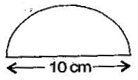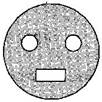### Perimeter and Area - Solutions 3

CBSE Class –VII Mathematics
NCERT Solutions
Chapter 11 Perimeter and Area (Ex. 11.3)

Question 1. Find the circumference of the circles with the following radius:
(a) 14 cm
(b) 28 mm
(c) 21 cm
Answer: (a) Circumference of the circle = $2\pi r$ = $2×\frac{22}{7}×14$ = 88 cm
(b) Circumference of the circle = $2\pi r$ = $2×\frac{22}{7}×28$ = 176 mm
(c) Circumference of the circle = $2\pi r$ = $2×\frac{22}{7}×21$ = 132 cm

Question 2. Find the area of the following circles, given that:
(b) diameter = 49 m
Answer: (a) Area of circle = $\pi {r}^{2}$ = $\frac{22}{7}×14×14$ = 22 x 2 x 14 = 616 mm2
(b) Diameter = 49 m
$\therefore$ radius = $\frac{49}{2}$ = 24.5 m
$\therefore$ Area of circle = $\pi {r}^{2}$ = $\frac{22}{7}×24.5×24.5$ = 22 x 3.5 x 24.5 = 1886.5 m2
(c) Area of circle = $\pi {r}^{2}$ = $\frac{22}{7}×5×5$ =  $\frac{550}{7}$ cm2

Question 3. If the circumference of a circular sheet is 154 m, find its radius. Also find the area of the sheet.
Answer: Circumference of the circular sheet = 154 m
$⇒$ $2\pi r$ = 154 m $⇒$ $r=\frac{154}{2\pi }$
$⇒$ $r=\frac{154×7}{2×22}$ = 24.5 m
Now, Area of circular sheet = $\pi {r}^{2}$ = $\frac{22}{7}×24.5×24.5$= 22 x 3.5 x 24.5 = 1886.5 m2
Thus, the radius and area of circular sheet are 24.5 m and 1886.5 m2 respectively.

Question 4. A gardener wants to fence a circular garden of diameter 21 m. Find the length of the rope he needs to purchase, if he makes 2 rounds of fence. Also, find the costs of the rope, if it cost Rs. 4 per meter.
Answer: Diameter of the circular garden = 21 m
$\therefore$ Radius of the circular garden = $\frac{21}{2}$ m
Now, Circumference of circular garden = $2\pi r$ = $2×\frac{22}{7}×\frac{21}{2}$ = 2 x 11 x 3 = 22 x 3 = 66 m
The gardener makes 2 rounds of fence so the total length of the rope of fencing
= 2 x $2\pi r$ = 2 x 66 = 132 m
Since the cost of 1 meter rope = Rs. 4
Therefore, cost of 132 meter rope = 4 x 132 = Rs. 528

Question 5. From a circular sheet of radius 4 cm, a circle of radius 3 cm is removed. Find the area of the remaining sheet.
Answer: Radius of circular sheet (R) = 4 cm and radius of removed circle (r) = 3 cm
Area of remaining sheet = Area of circular sheet – Area of removed circle
$\pi {\text{R}}^{2}-\pi {r}^{2}$ = $\pi \left({\text{R}}^{2}-{r}^{2}\right)$
$\pi \left({4}^{2}-{3}^{2}\right)$ = $\pi \left(16-9\right)$
= 3.14 x 7 = 21.98 cm2
Thus, the area of remaining sheet is 21.98 cm2.

Question 6. Saima wants to put a lace on the edge of a circular table cover of diameter 1.5 m. Find the length of the lace required and also find its cost if one meter of the lace costs Rs. 15.
Answer: Diameter of the circular table cover = 1.5 m
$\therefore$ Radius of the circular table cover = $\frac{1.5}{2}$ m
Circumference of circular table cover = $2\pi r$ = $2×3.14×\frac{1.5}{2}$ = 4.71 m
Therefore the length of required lace is 4.71 m.
Now the cost of 1 m lace = Rs. 15
Then the cost of 4.71 m lace = 15 x 4.71 = Rs. 70.65
Hence, the cost of 4.71 m lace is Rs. 70.65.

Question 7. Find the perimeter of the adjoining figure, which is a semicircle including its diameter.$\therefore$ Radius = $\frac{10}{2}$ = 5 cm
According to question,
Perimeter of figure = Circumference of semi-circle + diameter
$\pi r$ + D = $\frac{22}{7}×5+10$ = $\frac{110}{7}+10$
$\frac{110+70}{7}=\frac{180}{7}$ = 25.71 cm
Thus, the perimeter of the given figure is 25.71 cm.

Question 8. Find the cost of polishing a circular table-top of diameter 1.6 m, if the rate of polishing is Rs. 15/m2
Answer: Diameter of the circular table top = 1.6 m
$\therefore$ Radius of the circular table top = $\frac{1.6}{2}=$ 0.8 m
Area of circular table top = $\pi {r}^{2}$ = 3.14 x 0.8 x 0.8 = 2.0096 m2
Now cost of 1 m2 polishing = Rs. 15
Then cost of 2.0096 m2 polishing = 15 x 2.0096 = Rs. 30.14 (approx.)
Thus, the cost of polishing a circular table top is Rs. 30.14 (approx.)

Question 9. Shazli took a wire of length 44 cm and bent it into the shape of a circle. Find the radius of that circle. Also find its area. If the same wire is bent into the shape of a square, what will be the length of each of its sides? Which figure encloses more area, the circle or the square?
Answer: Total length of the wire = 44 cm
$\therefore$ the circumference of the circle = $2\pi r$ = 44 cm
$⇒$ $2×\frac{22}{7}×r=44$ $⇒$ $r=\frac{44×7}{2×22}$ = 7 cm
Now Area of the circle = $\pi {r}^{2}$ = $\frac{22}{7}×7×7$ = 154 cm2
Now the wire is converted into square.
Then perimeter of square = 44 cm
$⇒$ 4 x side = 44 $⇒$ side = $\frac{44}{4}$ = 11 cm
Now area of square = side x side = 11 x 11 = 121 cm2
Therefore, on comparing the area of circle is greater than that of square, so the circle enclosed more area.

Question 10. From a circular card sheet of radius 14 cm, two circles of radius 3.5 cm and a rectangle of length 3 cm and breadth 1 cm are removed (as shown in the adjoining figure). Find the area of the remaining sheet.Answer: Radius of circular sheet (R) = 14 cm and Radius of smaller circle $\left(r\right)$ = 3.5 cm
Length of rectangle $\left(l\right)$ = 3 cm and breadth of rectangle $\left(b\right)$ = 1 cm
According to question,
Area of remaining sheet = Area of circular sheet – (Area of two smaller circles + Area of rectangle)
$\pi {\text{R}}^{2}-\left[2\left(\pi {r}^{2}\right)+\left(l×b\right)\right]$
$\frac{22}{7}×14×14-\left[\left(2×\frac{22}{7}×3.5×3.5\right)-\left(3×1\right)\right]$
= 22 x 14 x 2 – [44 x 0.5 x 3.5 + 3]
= 616 – 80
= 536 cm2